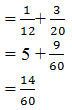New Student User - Use Code HELLO

# Mini Mock Test for SBI Clerk 2018 Quantitative Aptitude

## Question 1.

A borrowed Rs 830 from B at 12% for 3 years. He then added some amount of money to C at 14%. If A gain 3.90 in the whole transaction, how much he added from his side.

1) 105
2) 125
3) 98
4) 117
5) 130

## Question 2.

A man sold purchased worth Rs 400. He sold ¾ at a loss of 10% and the remaining at a gain of 10%. Find what % on whole he gets?
1) Loss of 7%
2) Gain of 7%
3) Loss of 5%
4) Gain of 5%
5) No loss no gain

## Question 3.

Even after reducing the market price of a transistor by Rs 32, a shopkeeper makes a profit of 15%, if the cost price be Rs 320, what percentage of profit would be have made if he had sold the transistor at the market price.
1) 17%
2) 21%
3) 25%
4) 29%
5) 33%

## Question 4.

A man wanted to distribute his money between his two sons (one is 15 year and another one is 13 years old) in such a manner that when they become 18 years old, they get same money. If he has Rs 3, 90, 300 and rate is 4%. Find the Principal amount?
1) 2, 02, 800 and 1, 87,500
2) 2, 00, 500 and 1, 89, 800
3) 2, 00, 005 and 1, 90, 295
4) 2, 10, 100 and 1, 80, 200
5) 2, 25, 000 and 1, 65, 300

## Question 5.

33 men can do a job in 30 days. If 44 men started the work together and after every day, one person leaves the work then what is the minimum no. of days required to complete the whole work.
1) 49
2) 53
3) 44
4) 35
5) 39

## Question 6.

A thief is spotted by policemen from a distance of 200 meters. When the policemen started to run after the thief, the thief also started running assuming that the speed of the thief to be 10 km and that of the policemen is 12 km/hr. How much will the thief have run before he caught by the policemen?
1) 0.5 km
2) 2 km
3) 4.5 km
4) 1 km
5) 6 km

## Question 7.

A and B can do a work in 12 days. While B+C can do it in 6 2/3 days. Work to be completed by A, B and C in 3, 4, and 7 days. Find how many days A alone would complete the work.
1) 27 days
2) 24 days
3) 30 days
4) 37 days
5) 33 days

## Question 8.

A monkey climb a pole of 60 cm height. It climbs 5 cm above in 1st minute and 4 cm down in 2nd minute. In how many minutes monkey will climb on the pole?
1) 110 minute
2) 107 minute
3) 103 minute
4) 115 minute
5) 111 minute

## Question 9.

An electronic contactor has a certain length of wire. If 10% of wire has been stolen and 70% of the remaining was sold out. Find the original length of wire if 810-meter wire still left.
1) 2850 meter
2) 3000 meter
3) 3125 meter
4) 2652 meter
5) 3200 meter

## Question 10.

Two trains running at 45 km/ hr and 36 km/ph respectively. On parallel lines in an opposite direction, it is observed that both pass each other in 8 seconds and when they are running in the same direction at the same rate as before, a person sitting in the faster train observes that he passes the other in 30 seconds. Find the length of the trains?
1) 93 metre
2) 105 metre
3) 97 meter
4) 109 metre
5) 113 meter

## Solutions

### Ans 1.  105X = 105

### Ans 2. Loss of 5### Ans 4. 2, 02, 800 and 1, 07,500

15 years old get 33-year interest
13 years old get 5 years interest
Difference of interest get = 5-3 = 2 Convert ratio into percentage = 4%
Here, Assume 1 is interest and 25 is principal amount
So, compound interest = 25 + 1 = 26### Ans 7. 30 days

A +B = 12
B +C =6 2/3
A +B +C’s total work togetherTotal 60 units and 1 day’s work is units
3A + 4B + 7C = 60 units
3A + 3B + 1B + 1C + 6C = 60 units
3(A + B) + 1(B + C) + 6C = 60 units
3(5) + 1(9) + 6C = 60
15 + 9 +6C = 60
6C = 60 – 24
6C = 36
C = 6
A = 2, B = 3, C = 6
A’s 1 day work = 2 units
A’s total work in how many days = 60 units### Ans 8. 111 minutes

1st minute a monkey will climb 5 cm and in 2nd minute it come 4 cm down.
So, in 1st cycle monkey cover 1 cm.
1st cycle of 2 minute = 1 cm
1cm          2 minute
55 cm          ?
110 minute
In the next 1 minute, monkey will cover 5 cm. So, it takes 111 minutes to climb 60 cm pole.

### Ans 10. 105 meter